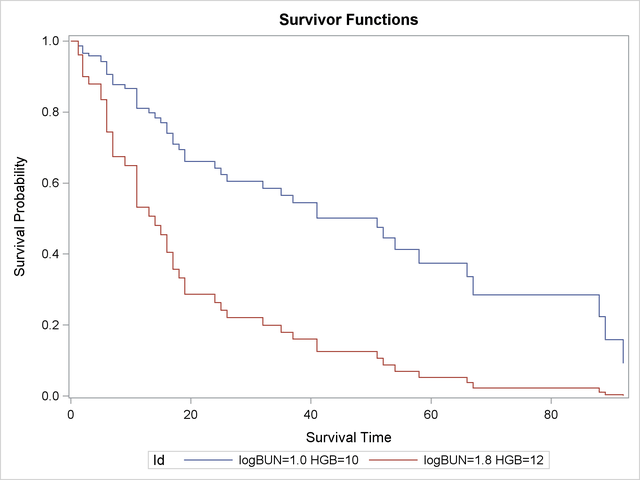## Example 66.8 Survivor Function Estimates for Specific Covariate Values

You might want to use your regression analysis results to generate predicted survival curves for subjects not in the study. The COVARIATES= data set in the BASELINE statement enables you to specify the sets of covariate values for the prediction. By using the PLOTS= option in the PROC PHREG statement, you can display a survival curve for each row of covariates in the COVARIATES= data set. You can elect to output the predicted survival curves in a SAS data set by using just the BASELINE statement. This example illustrates these two tasks by using the Myeloma data in Example 66.1.

In Example 66.1, variables LogBUN and HGB were identified as the most important prognostic factors for the myeloma data. Two sets of covariates for predicting the survivor function are saved in the data set Inrisks in the following DATA step. Also created in this data set is the variable Id, whose values will be used in identifying the covariate sets in the survival plot.

```data Inrisks;
length Id \$20;
input LogBUN HGB Id \$12-31;
datalines;
1.00 10.0  logBUN=1.0 HGB=10
1.80 12.0  logBUN=1.8 HGB=12
;
```

The following statements produce the plot in Output 66.8.1 and create the BASELINE data set Pred1:

```ods graphics on;
proc phreg data=Myeloma plots(overlay)=survival;
model Time*VStatus(0)=LogBUN HGB;
baseline covariates=Inrisks out=Pred1 survival=_all_ / rowid=Id;
run;
ods graphics off;
```

The COVARIATES= option in the BASELINE statement specifies the data set that contains the set of covariates of interest. The PLOTS= option in the PROC PHREG statement creates the survivor plot. The OVERLAY suboption overlays the two curves in the same plot. If the OVERLAY suboption is not specified, each curve is displayed in a separate plot. The ROWID= option in the BASELINE statement specifies that the values of the variable Id in the COVARIATES= data set be used to identify the curves in the plot. The SURVIVAL=_ALL_ option in the BASELINE statement requests that the estimated survivor function, standard error, and lower and upper confidence limits for the survivor function be output into the SAS data set specified in the OUT= option.

The survival Plot (Output 66.8.1) contains two curves, one for each of row of covariates in the data set Inrisks.

Output 66.8.1 Estimated Survivor Function PlotFinally, PROC PRINT is used to print out the observations in the data set Pred1 for the realization LogBUN=1.00 and HGB=10.0:

```proc print data=Pred1(where=(logBUN=1 and HGB=10));
run;
```

As shown in Output 66.8.2, there are 32 observations representing the survivor function for the realization LogBUN=1.00 and HGB=10.0. The first observation has survival time 0 and survivor function estimate 1.0. Each of the remaining 31 observations represents a distinct event time in the input data set Myeloma. These observations are presented in ascending order of the event times. Note that all the variables in the COVARIATE=InRisks data set are included in the OUT=Pred1 data set. Likewise, you can print out the observations that represent the survivor function for the realization LogBUN=1.80 and HGB=12.0.

Output 66.8.2 Survivor Function Estimates for LogBUN=1.0 and HGB=10.0
Obs Id LogBUN HGB Time Survival StdErrSurvival LowerSurvival UpperSurvival
1 logBUN=1.0 HGB=10 1 10 0.00 1.00000 . . .
2 logBUN=1.0 HGB=10 1 10 1.25 0.98678 0.01043 0.96655 1.00000
3 logBUN=1.0 HGB=10 1 10 2.00 0.96559 0.01907 0.92892 1.00000
4 logBUN=1.0 HGB=10 1 10 3.00 0.95818 0.02180 0.91638 1.00000
5 logBUN=1.0 HGB=10 1 10 5.00 0.94188 0.02747 0.88955 0.99729
6 logBUN=1.0 HGB=10 1 10 6.00 0.90635 0.03796 0.83492 0.98389
7 logBUN=1.0 HGB=10 1 10 7.00 0.87742 0.04535 0.79290 0.97096
8 logBUN=1.0 HGB=10 1 10 9.00 0.86646 0.04801 0.77729 0.96585
9 logBUN=1.0 HGB=10 1 10 11.00 0.81084 0.05976 0.70178 0.93686
10 logBUN=1.0 HGB=10 1 10 13.00 0.79800 0.06238 0.68464 0.93012
11 logBUN=1.0 HGB=10 1 10 14.00 0.78384 0.06515 0.66601 0.92251
12 logBUN=1.0 HGB=10 1 10 15.00 0.76965 0.06779 0.64762 0.91467
13 logBUN=1.0 HGB=10 1 10 16.00 0.74071 0.07269 0.61110 0.89781
14 logBUN=1.0 HGB=10 1 10 17.00 0.71005 0.07760 0.57315 0.87966
15 logBUN=1.0 HGB=10 1 10 18.00 0.69392 0.07998 0.55360 0.86980
16 logBUN=1.0 HGB=10 1 10 19.00 0.66062 0.08442 0.51425 0.84865
17 logBUN=1.0 HGB=10 1 10 24.00 0.64210 0.08691 0.49248 0.83717
18 logBUN=1.0 HGB=10 1 10 25.00 0.62360 0.08921 0.47112 0.82542
19 logBUN=1.0 HGB=10 1 10 26.00 0.60523 0.09136 0.45023 0.81359
20 logBUN=1.0 HGB=10 1 10 32.00 0.58549 0.09371 0.42784 0.80122
21 logBUN=1.0 HGB=10 1 10 35.00 0.56534 0.09593 0.40539 0.78840
22 logBUN=1.0 HGB=10 1 10 37.00 0.54465 0.09816 0.38257 0.77542
23 logBUN=1.0 HGB=10 1 10 41.00 0.50178 0.10166 0.33733 0.74639
24 logBUN=1.0 HGB=10 1 10 51.00 0.47546 0.10368 0.31009 0.72901
25 logBUN=1.0 HGB=10 1 10 52.00 0.44510 0.10522 0.28006 0.70741
26 logBUN=1.0 HGB=10 1 10 54.00 0.41266 0.10689 0.24837 0.68560
27 logBUN=1.0 HGB=10 1 10 58.00 0.37465 0.10891 0.21192 0.66232
28 logBUN=1.0 HGB=10 1 10 66.00 0.33626 0.10980 0.17731 0.63772
29 logBUN=1.0 HGB=10 1 10 67.00 0.28529 0.11029 0.13372 0.60864
30 logBUN=1.0 HGB=10 1 10 88.00 0.22412 0.10928 0.08619 0.58282
31 logBUN=1.0 HGB=10 1 10 89.00 0.15864 0.10317 0.04435 0.56750
32 logBUN=1.0 HGB=10 1 10 92.00 0.09180 0.08545 0.01481 0.56907